Updated by Jon Samuelson on Nov 04, 2019REPORTJon Samuelson
Owner
13 items   8 followers   0 votes   6.71k views

# Elementary Math Websites To Practice Fractions

A list developed by Cindy Lawrence, 3rd grade teacher in Texas. This list accompanies Unit 8 of the Third Grade Everyday Math Curriculum.

1

## ABCya! Elementary Math - Fraction Tiles - Virtual ManipulativesABCya! Fraction tiles are a good way for students to explore and understand how parts make up a whole. Teachers and parents can utilize this resource when giving a lesson on fractions with students.

2

## Math Games: Fractions Tutorial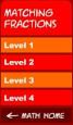Fractions Tutorial. Fun free flash games teach logical thinking and math skills.

3

## Math Games: Matching Fractions Level One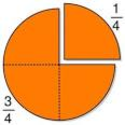Matching game - match the picture of the fraction to the fraction.

4

## Math Games: Mixed Fractions Level 1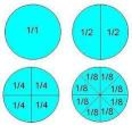Math matching game to learn mixed fractions.

5

## Math Games: Matching Equivalent Fraction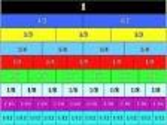Math matching game to learn equivalent fractions.

6

## Math Games:Balloon Pop Fractions Level 1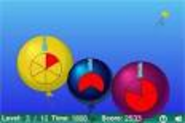Balloon Pop Fractions level 1. Fun free flash math game teaches logical thinking and mental math.

7

## Math Games: Reduce Fractions Shoot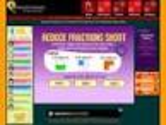Practice reducing fractions in this fun free arcade math game.

8

## Fractions Shoot Math GameLearn about simple fractions. Equal, unequal, Halves, Thirds, Fourths with this fun math arcade game

9

## Math Games: Fractions as Parts of a Set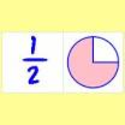Matching game to learn fractions as parts of a set.

10

## Math Games: Convert Fractions to Decimals - Fruit Shoot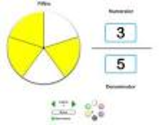Practice converting fractions to decimals in this fun free arcade flash math game.

11

## Escape from Fraction ManorAction packed math site for elementary and middle school students featuring math games, math word problems, math worksheets, logic puzzles, and math videos.

12

## BBC - Schools - Fractions activity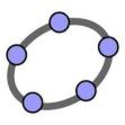An animated KS2 activity for the Maths topic: Fractions

13

## Tony's Pizza Fraction | MrNussbaum.com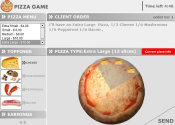Order different types of pizza in fraction forms to create pizzas correctly.